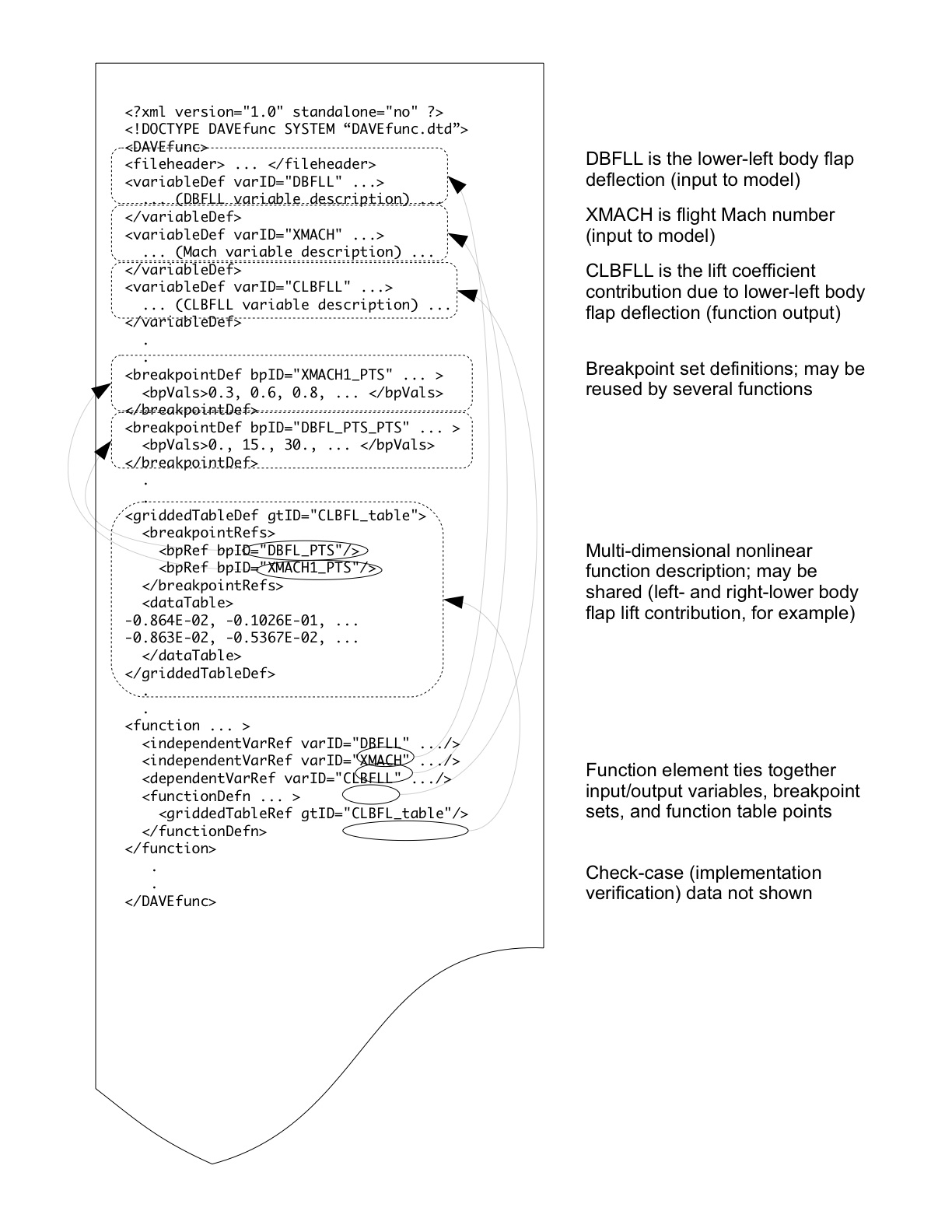### 6.1. The `DAVEfunc` major element

The `DAVEfunc` element contains both data tables and equations for a particular static model. A `DAVEfunc` element is broken into six components: a file header, variable definitions, breakpoint definitions, table definitions, function definitions and optional check-cases. This decomposition reflects common practice in engineering development flight-simulation models in which the aerodynamic database is usually captured in multi-dimensional, linearly interpolated function tables. The inputs to these tables are usually state variables of the simulation (such as Mach number or angle-of-attack). The outputs from these interpolated tables are combined to represent forces and moments acting on the vehicle due to aerodynamics.

It is possible, using `DAVEfunc` and MathML-2 elements, to completely define an aerodynamic model without use of function tables (by mathematical combinations of input variables, such as a polynomial model) but this is not yet common in the American flight-simulation industry.

A `fileHeader` element is included to give background and reference data for the represented model.

Variables, or more properly signals, are used to route inputs and calculations through the subsystem model into outputs. Each variable is defined with a `variableDef` element. Variables can be thought of as parameters in a computer program or signal paths on a block diagram. They can be inputs to the subsystem model, constant values, outputs from the model, and/or the results of intermediate calculations. Variables must be defined for each input and output of any function element as well as any input or output of the subsystem represented. MathML-2 content markup can be used to define constant, intermediate, or output variables as mathematical combination of constant values, function table outputs, and other variables, but any presentation markup is not required and should be ignored by the processing application (except as required to generate documentation). Variables also represent the current value of a function (the `dependentVariableDef` in a `function` definition) so the output of functions can be used as inputs to other variables or functions.

Breakpoint definitions, captured in `breakpointDef` elements, consist of a list of monotonically increasing floating-point values separated by commas or white space. These sets are referenced by "gridded" function table definitions and may be referenced by more than one `function` definition.

Function table definitions, described by `griddedTableDef` and `ungriddedTableDef` elements, generally contain the bulk of data points in an aerodynamics model, and typically represent a smooth hyper-surface representing the value of some aerodynamic non-dimensional coefficient as a function of one or more vehicle states (typically Mach number, angle-of-attack, control surface deflection, and/or angular body rates). These function tables can be either "gridded," meaning the function has a value at every intersection of each dimension's breakpoint, or "ungridded," meaning each data point has a specified coordinate location in n-space. The same table can be reused in several functions, such as a left- and right-aileron moment contribution.

Function definitions (described by `function` elements) connect breakpoint sets and data tables to define how an output signal (or dependent variable) should vary with one or more input signals (or independent variables). The valid ranges of input-signal magnitudes, along with extrapolation requirements for out-of-range inputs, can be defined. There is no limit to the number of independent variables, or function dimensionality, of the function.

Check-case data (described by a single `checkData` element) can be included to provide information to automatically verify the proper implementation of the model by the recipient. Multiple check-cases can (and should) be specified using multiple `staticShot` test-case definitions, as well as optional internal signal values within the model to assist in debugging an instantiation of the model by the recipient.

Figure 1 contains excerpts from an example model, showing five of the six major parts of a DAVE-ML file.

Figure 1.  Excerpts from an example DAVE-ML fileA simpler version of a `function` is available in which the dependent variable breakpoint values and dependent output values are specified directly inside the `function` body. This may be preferred for models that do not reuse function or breakpoint data.

A third form of `function` is to give the gridded table values or ungridded table values inside the `function` body, but refer to externally defined breakpoint sets. This allows reuse of the breakpoint sets by other functions but keeps the table data private.

2011-03-31